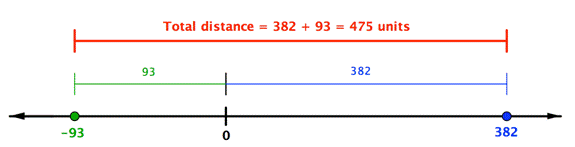## Adding and Subtracting Real Numbers

### Learning Outcomes

• Add and subtract real numbers
• Add real numbers with the same and different signs
• Subtract real numbers with the same and different signs
• Simplify combinations that require both addition and subtraction of real numbers.

Some important terminology to remember before we begin is as follows:

• integers: counting numbers like  $1, 2, 3$, etc., including negatives and zero
• real number: fractions, negative numbers, decimals, integers, and zero are all real numbers
• absolute value: a number’s distance from zero; it’s always positive. $|-7| = 7$
• sign: this refers to whether a number is positive or negative, we use $+$ for positive (to the right of zero on the number line) and $-$ for negative (to the left of zero on the number line)
• difference: the result of subtraction
• sum: the result of addition

The ability to work comfortably with negative numbers is essential to success in algebra. For this reason we will do a quick review of adding, subtracting, multiplying and dividing integers. Integers are all the positive whole numbers, zero, and their opposites (negatives). As this is intended to be a review of integers, the descriptions and examples will not be as detailed as a normal lesson.

When adding integers we have two cases to consider. The first case is whether the signs match (both positive or both negative). If the signs match, we will add the numbers together and keep the sign.

If the signs don’t match (one positive and one negative number) we will subtract the numbers (as if they were all positive) and then use the sign from the larger number. This means if the larger number is positive, the answer is positive. If the larger number is negative, the answer is negative.

### To add two numbers with the same sign (both positive or both negative)

• Add their absolute values (without the $+$ or $-$ sign)
• Give the sum the same sign.

### To add two numbers with different signs (one positive and one negative)

• Find the difference of their absolute values. (Note that when you find the difference of the absolute values, you always subtract the lesser absolute value from the greater one.)
• Give the sum the same sign as the number with the greater absolute value.

### Example

Find $23–73$.

Another way to think about subtracting is to think about the distance between the two numbers on the number line. In the example below, $382$ is to the right of 0 by $382$ units, and $−93$ is to the left of 0 by 93 units. The distance between them is the sum of their distances to 0: $382+93$.### Example

Find $382–\left(−93\right)$.

The following video explains how to subtract two signed integers.

### Example

Find $-\frac{3}{7}-\frac{6}{7}+\frac{2}{7}$

### Try It

In the following video you will see an example of how to add three fractions with a common denominator that have different signs.

### Example

Evaluate $27.832+(−3.06)$. When you add decimals, remember to line up the decimal points so you are adding tenths to tenths, hundredths to hundredths, and so on.

### Try It

In the following video are examples of adding and subtracting decimals with different signs.

## Contribute!

Did you have an idea for improving this content? We’d love your input.